## How to Graph Quadratic Functions

Putting quadratic functions on a coordinate plane is the process of quadratic graphing functions.
This guide will teach you how to draw graphs of quadratic functions. Both general form and vertex form can be used to graph quadratic functions.

### How to draw graphs of quadratic functions, step by step

Graphing quadratic functions is a way to look at the nature of quadratic functions in a visual way. The coefficient $$a$$ of the quadratic function $$f(x) \ = \ ax^2 \ + \ bx \ + \ c$$, where $$a, \ b,$$, and $$c$$ are real numbers and $$a \ ≠ \ 0$$, determines the shape of the parabola, which is the graph of a quadratic function.

A quadratic function's vertex form is $$f(x) \ = \ a(x \ - \ h)^2 \ +\ k$$, where $$(h,k)$$ is the parabola's vertex. The coefficient of a quadratic function tells whether the graph goes up or down.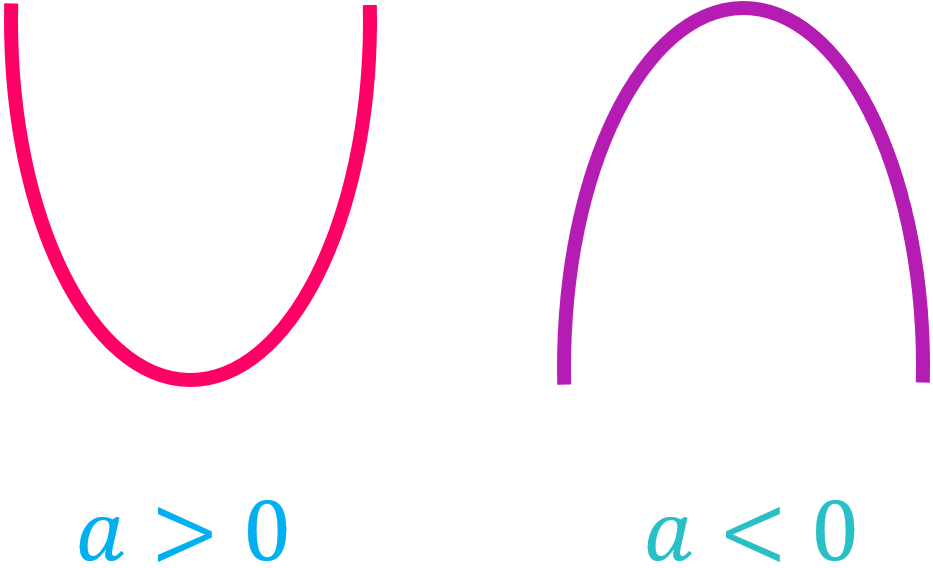Note that the coefficient $$a$$ also controls the rate at which the graph of the quadratic function goes up (or down) from the vertex. If $$a$$ is bigger and positive, the process goes up more quickly, and the graph looks thinner.

### Using vertex form to draw graphs of quadratic functions

We will learn how to plot each quadratic function step by step. Think about the general quadratic function $$f(x) \ = \ ax^2 \ + \ bx \ + \ c$$.

First, we rearrange it so that $$f(x) \ = \ ax^2 \ + \ bx \ + \ c$$ becomes $$f(x) \ = \ a(x \ + \ \frac{b}{2a})^2 \ - \ \frac{Δ}{4a}$$. The discriminant is the term $$Δ$$, which is given by $$Δ \ = \ b^2 \ - \ 4ac$$.

Here, the point where the parabola starts and ends is $$(h \ , \ k) \ = \ (-\frac{b}{2a} \ , \ -\frac{Δ}{4a})$$. Now, to draw the graph of $$f(x)$$, we start with the graph of $$x^2$$ and change it in several ways:

Step 1: Change $$x^2$$ to $$ax^2$$. This is the original parabola's vertical scale. If $$a$$ is negative, the mouth of the parabola will also change from positive to negative. How much the scaling goes up or down depends on how big $$a$$ is.

Consider this graph as $$x^2$$: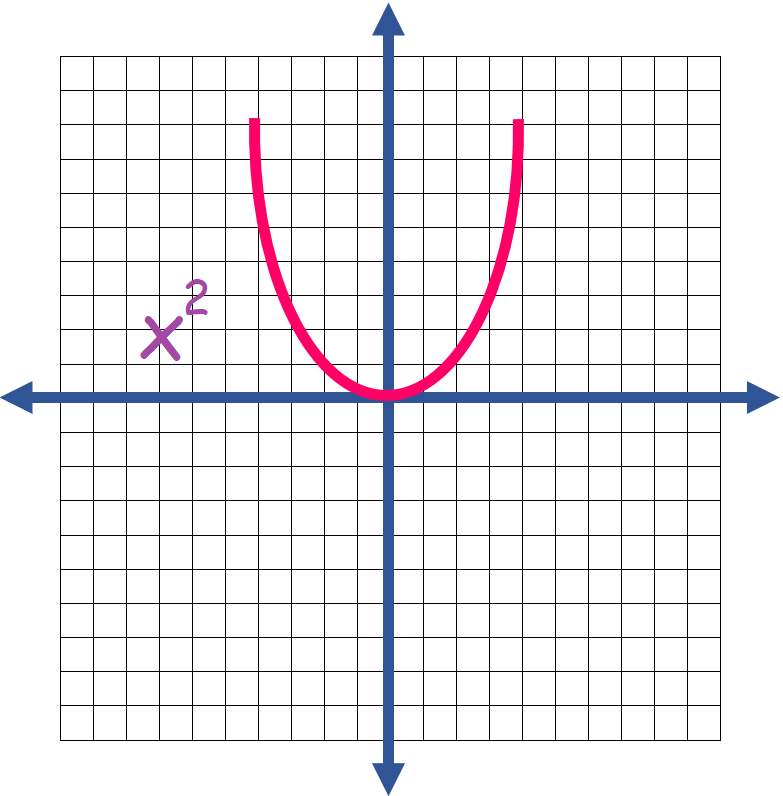If $$a \ > \ 0$$, $$ax^2$$ becomes like this: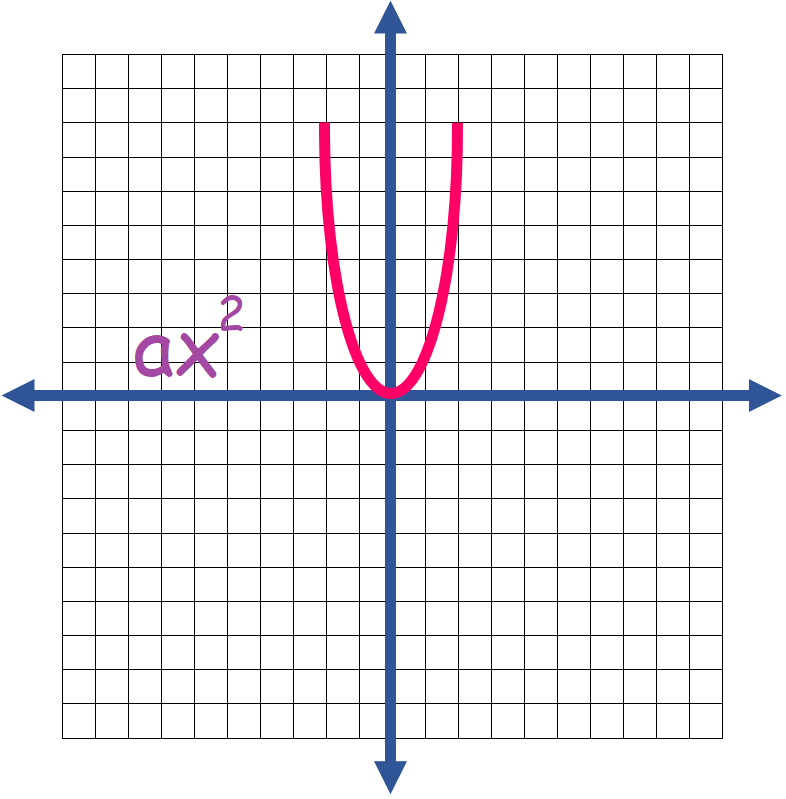But if $$a \ < \ 0$$, $$ax^2$$ becomes like this: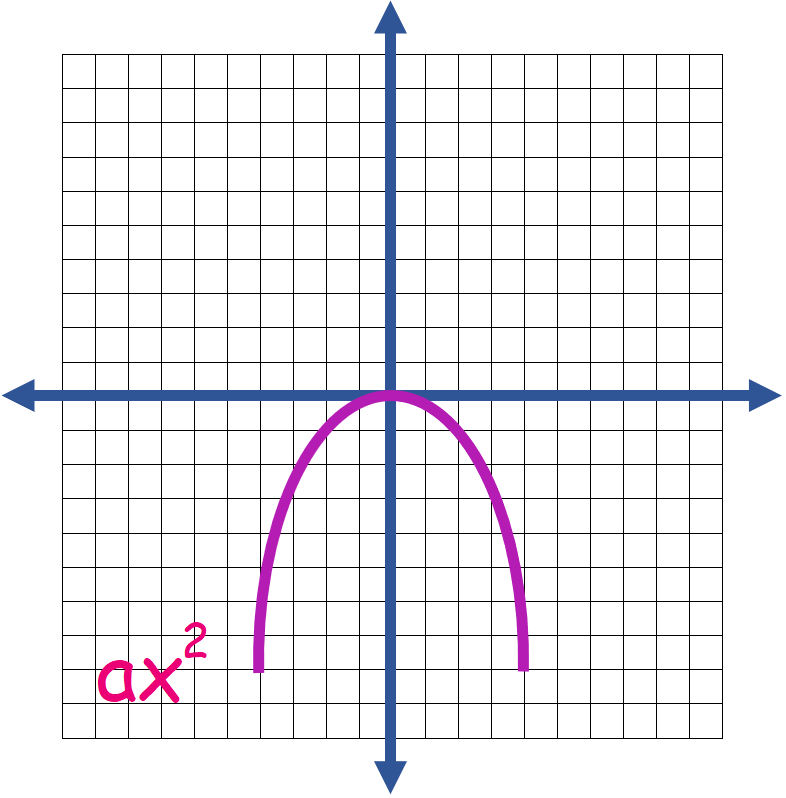Step 2: Change $$ax^2$$ to a(x \ + \ \frac{b}{2a})^2. This is a horizontal shift of $$|\frac{b}{2a}|$$ units. The sign of $$\frac{b}{2a}$$ will show which way the shift will go. The new point of the parabola's vertex will be $$(\frac{b}{2a} \ , \ 0)$$. The figure below shows an example of a shift:

If $$\frac{b}{2a} \ = \ -2$$: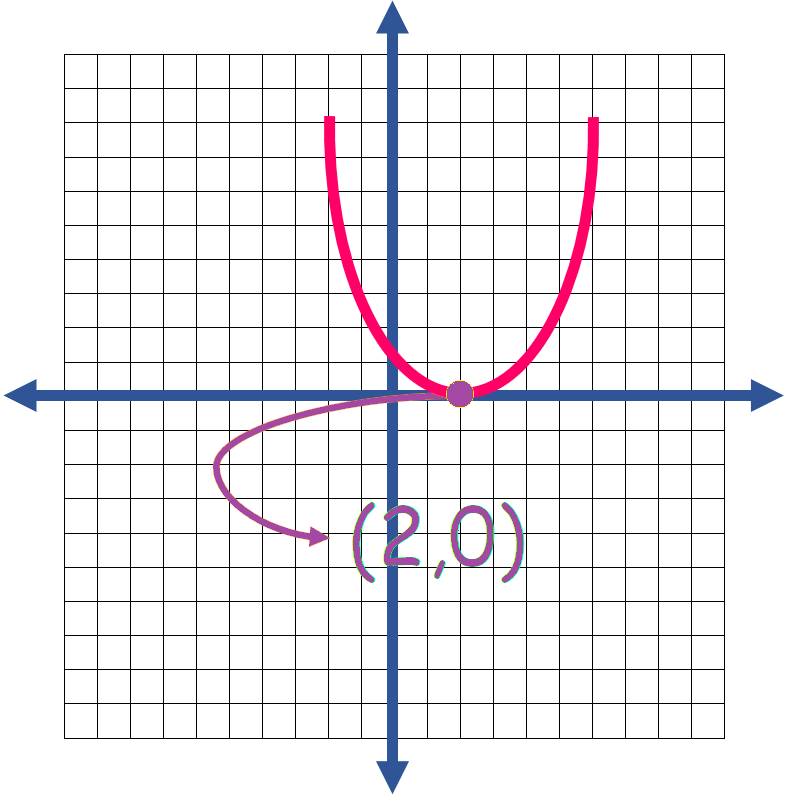Step 3: Change $$a(x \ + \ \frac{b}{2a})^2$$ to $$a(x \ + \ \frac{b}{2a})^2 \ - \ \frac{Δ}{4a}$$ . This is a vertical shift by $$|\frac{Δ}{4a}|$$ units. The sign of $$\frac{Δ}{4a}$$ will show which way the shift will go. The point where the parabola ends will be at $$(-\frac{b}{2a} \ , \ -\frac{Δ}{4a})$$. The figure below shows an example of a shift:

If $$\frac{b}{2a} \ = \ -3 \ , \ \frac{Δ}{4a} \ = \ 4$$: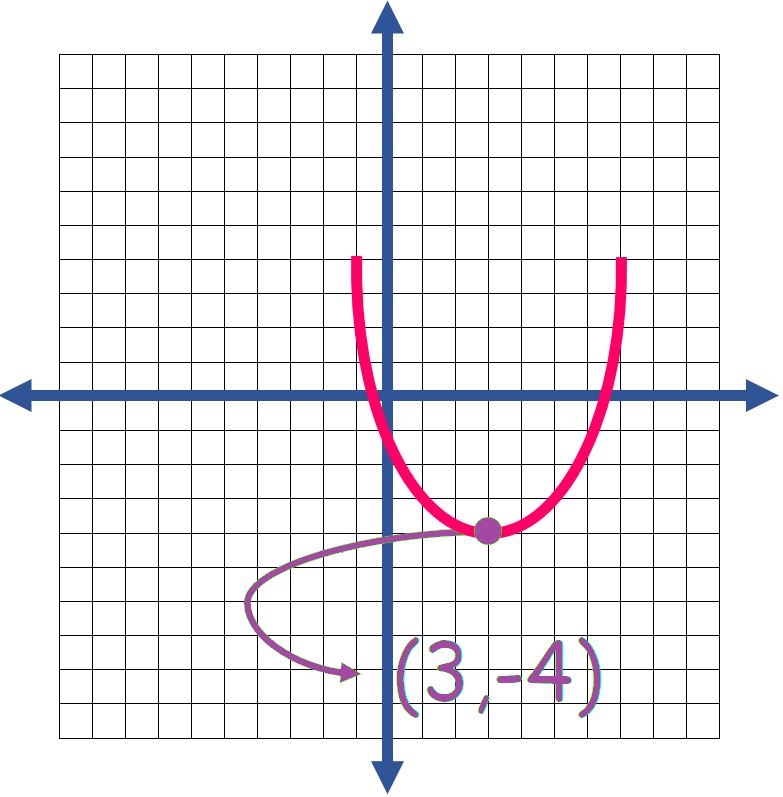### Using standard form to draw graphs of quadratic functions

$$f(x) \ = \ ax^2 \ + \ bx \ + \ c$$ is the general equation for a quadratic function. Using the standard form of the function, we can convert the general form to the vertex form, then draw the quadratic function diagram, or find the axis of symmetry and y-intercept of the graph and draw it.

### Exercises for Graphing Quadratic Functions

1) Sketch the graph: $$x^2 \ - \ 3$$

2) Sketch the graph: $$x^2 \ + \ 1$$

3) Sketch the graph: $$(x \ - \ 2)^2 \ + \ 1$$

4) Sketch the graph: $$(x \ + \ 3)^2 \ - \ 3$$

5) Sketch the graph: $$(x \ + \ 2.5)^2 \ - \ 4$$

6) Sketch the graph: $$(x \ - \ 1)^2 \ - \ 5$$

7) Sketch the graph: $$x^2 \ + \ 4$$

8) Sketch the graph: $$(x \ - \ 2)^2 \ + \ 3$$

9) Sketch the graph: $$(x \ + \ 3)^2 \ + \ 4$$

10) Sketch the graph: $$(x \ + \ 2)^2 \ - \ 5$$

1) Sketch the graph: $$x^2 \ - \ 3$$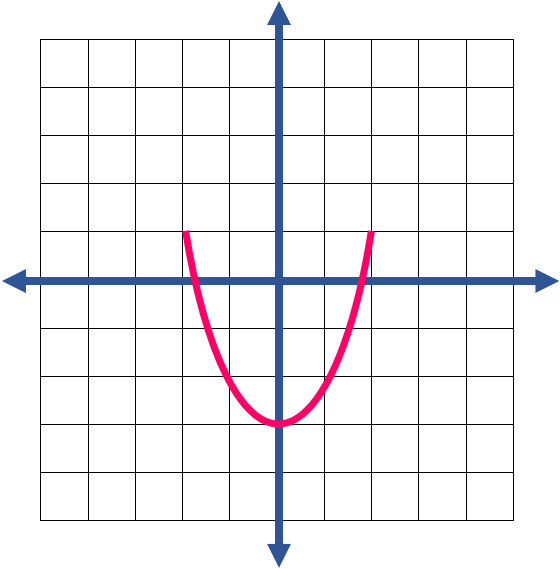2) Sketch the graph: $$x^2 \ + \ 1$$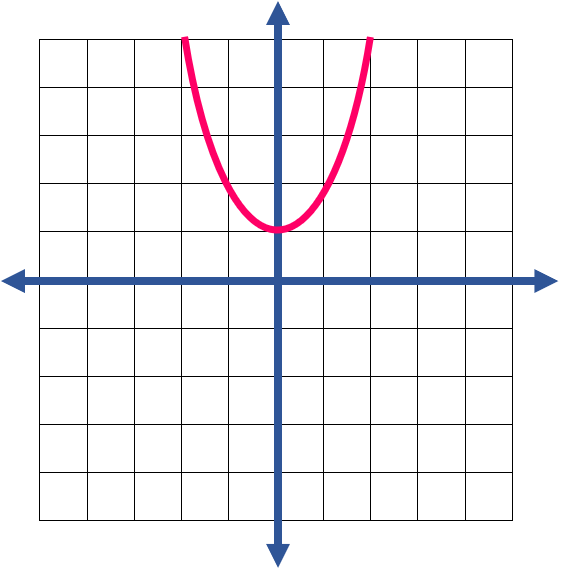3) Sketch the graph: $$(x \ - \ 2)^2 \ + \ 1$$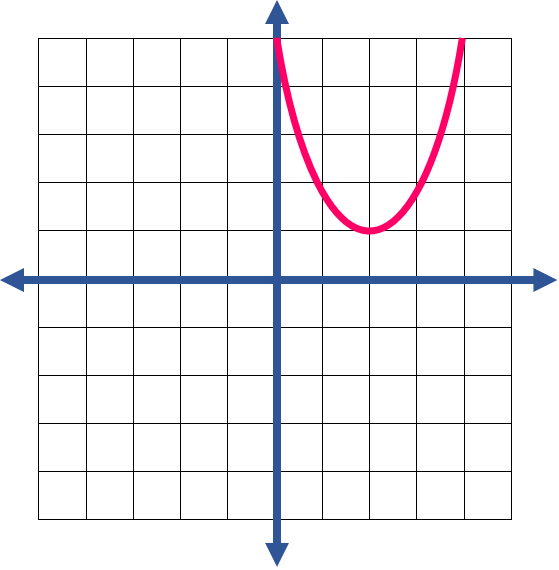4) Sketch the graph: $$(x \ + \ 3)^2 \ - \ 3$$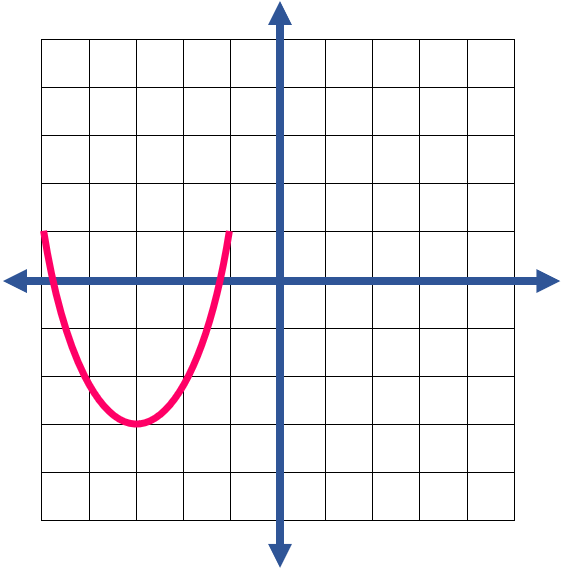5) Sketch the graph: $$(x \ + \ 2.5)^2 \ - \ 4$$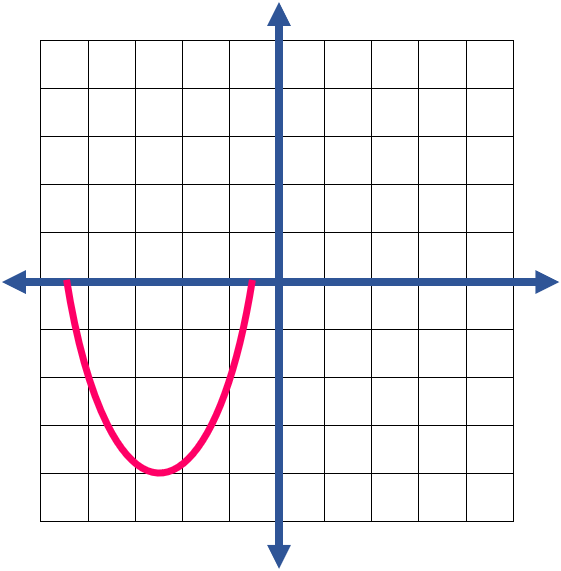6) Sketch the graph: $$(x \ - \ 1)^2 \ - \ 5$$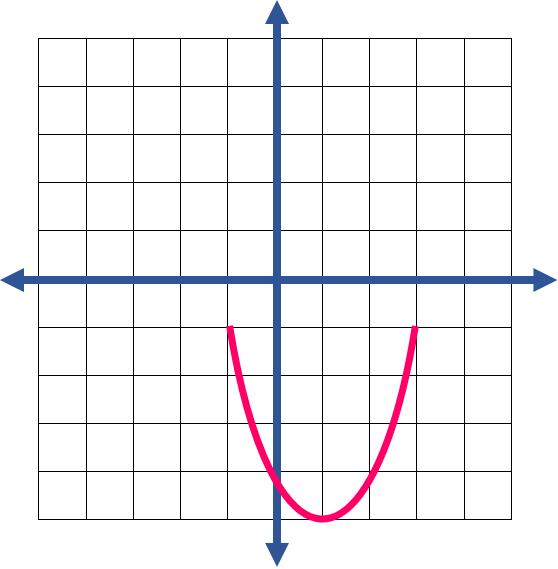7) Sketch the graph: $$x^2 \ + \ 4$$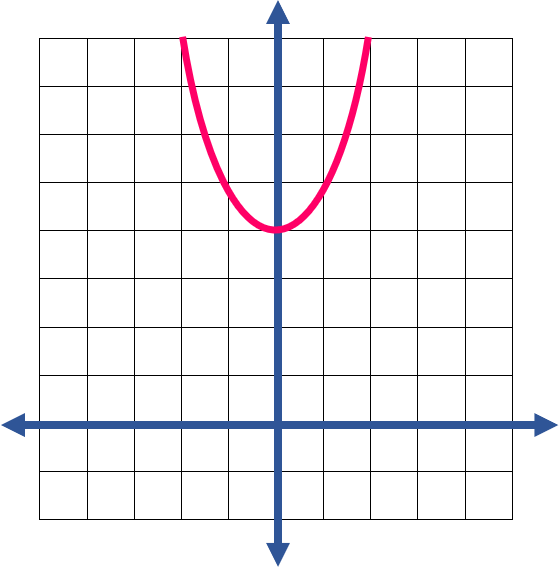8) Sketch the graph: $$(x \ - \ 2)^2 \ + \ 3$$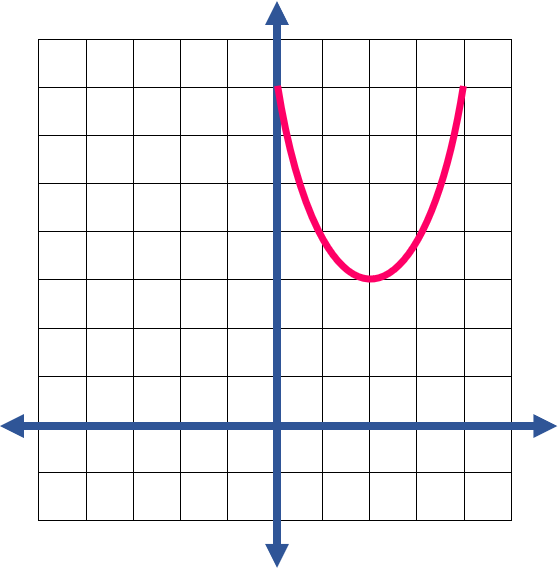9) Sketch the graph: $$(x \ + \ 3)^2 \ + \ 4$$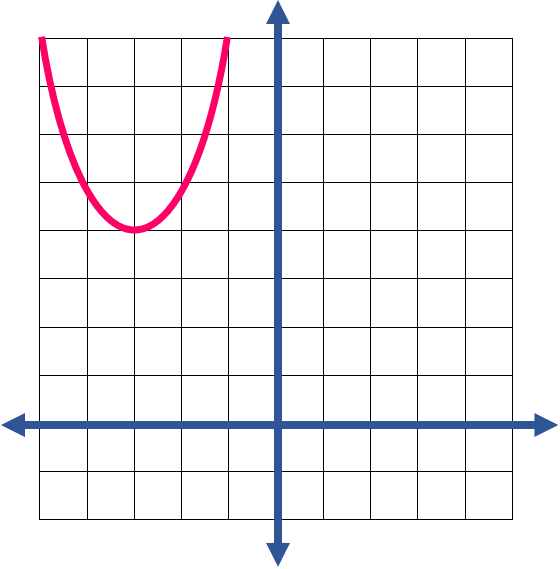10) Sketch the graph: $$(x \ + \ 2)^2 \ - \ 5$$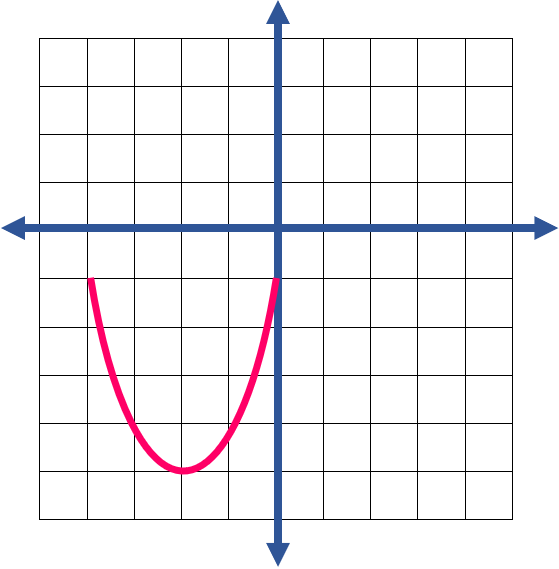## Graphing Quadratic Functions Practice Quiz

### New releases

$6.99$4.99

### TSI Math for Beginners 2022

$24.99$14.99

### The Most Comprehensive Accuplacer Math Preparation Bundle

$76.99$33.99

### The Most Comprehensive ALEKS Math Preparation Bundle

$76.99$34.99